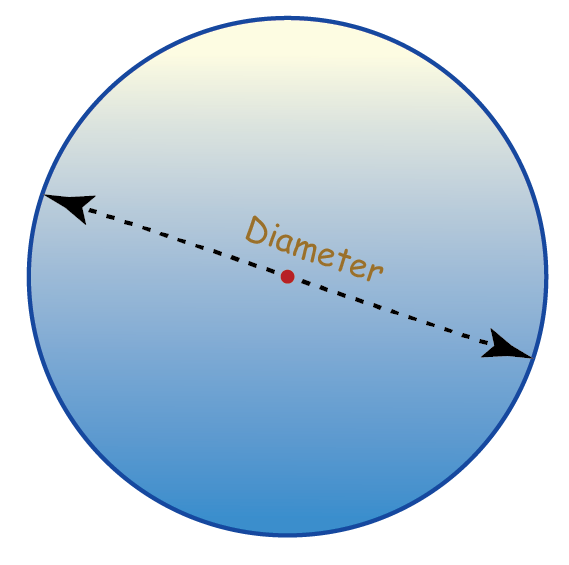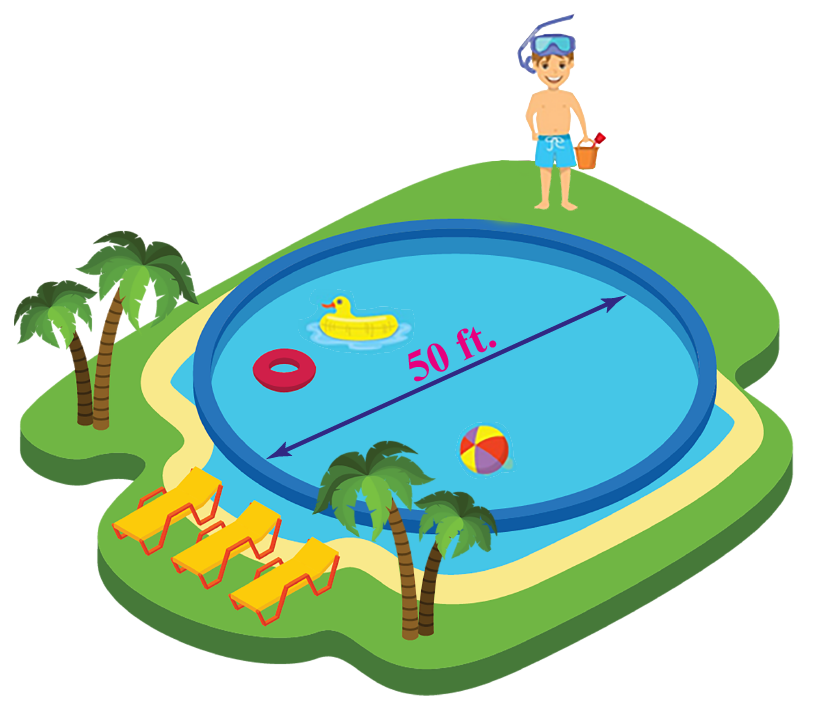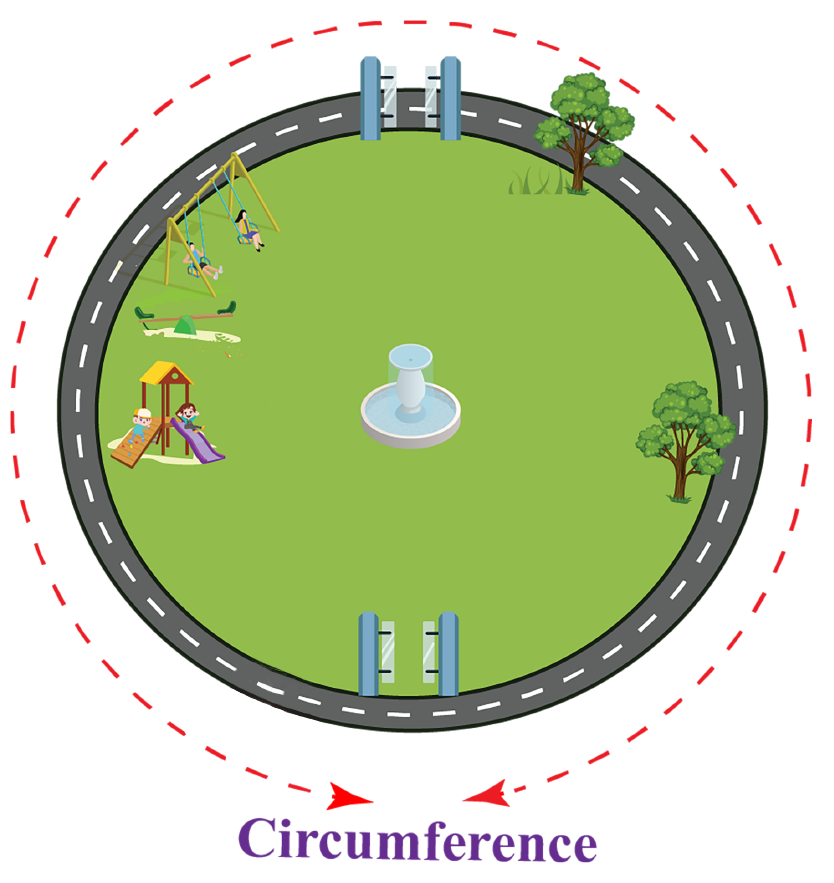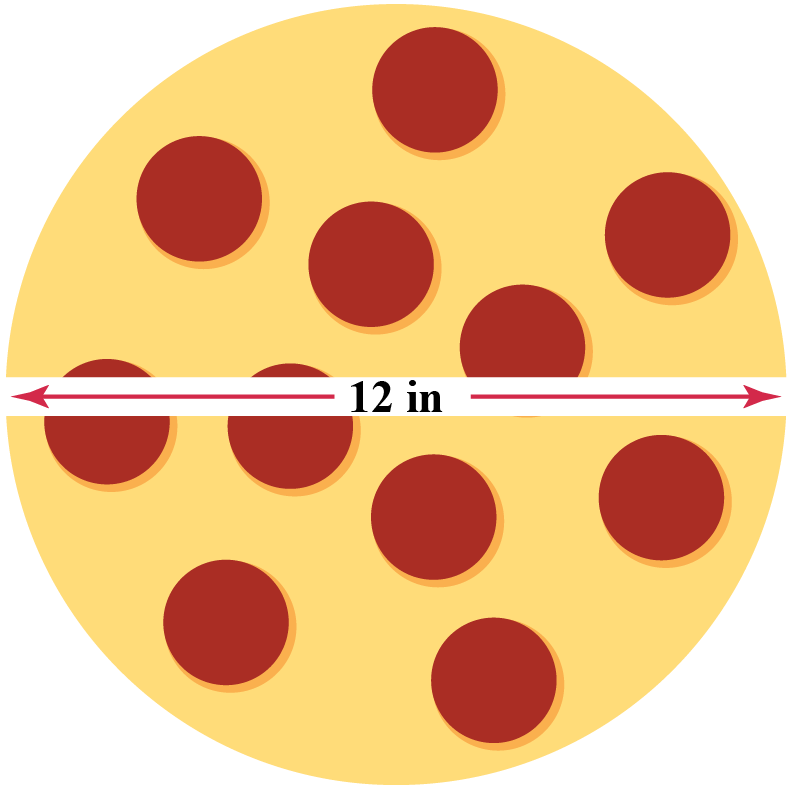# Circumference to Diameter

Circumference to Diameter

We know that the circle is a collection of points in which all the points are equidistant from the center point $$O$$.

The circumference of a circle is its boundary or the length of the complete one of a circle.

The diameter of a circle is a straight line joining a point from one end of the circle to a point on the other end of the circle, passing through the center.In this short article, we will learn about the relationship between the circumference and the diameter of the circles.

## Lesson Plan

 1 What Is Meant by Circumference To Diameter? 4 Important Notes on Circumference From Diameter 5 Solved Examples on Circumference From Diameter 6 Thinking Out of the Box! 7 Practice Questions on Circumference From Diameter

## What Is Meant by Circumference To Diameter?

### Meaning of Circumference To Diameter

The circumference of a circle is related to its diameter.

If you know the diameter $$d$$ of a circle, then you can easily find the circumference $$C$$ using the relation: $$C=\pi d$$.

So, when the circumference $$C$$ is placed in ratio with the diameter $$d$$, The answer we get is $$\pi$$.

In fact, here comes the standard definition of $$\pi$$ as the ratio of circumference and diameter of a circle.

## What Is the General Formula To Calculate Circumference To Diameter?

### Formula for Circumference

The circumference of a circle can be calculated using the radius $$r$$ of the circle and the value of $$\pi$$.

 Circumference of the circle = $$2 \pi r$$

While using this formula, if we do not have the value of the radius, we can find it using the diameter.

That is, if the diameter is known, it can be divided by 2 to obtain the value of the radius because:

 Diameter of the circle = $$2r$$

Another way to calculate the circumference of a circle is by using the formula:

 Circumference of the circle = $$\pi \times$$ Diameter

## How To Use Formula To Calculate Circumference From Diameter?

To calculate the circumference of a circular shape when the diameter is given we need to use the formula for circumference.

$\text{Circumference}=\pi\times d$

Let's understand the concept with the help of an example.

Jason wants to paint the outer boundary of a circular swimming pool.

He knows that the diameter of the pool is $$50\mathrm{\;feet}$$.

To calculate the amount of paint required he needs the circumference of the pool.Let's help him to calculate the circumference of the pool.

Use the formula for circumference $$\text{Circumference}=\pi\times d$$ where $$d$$ is the diameter of the pool.

\begin{aligned}\text{Circumference}&=\pi\times d\\&=\pi\times 50\\&=50\end{aligned}

Therefore the circumference of the pool is $$50\pi$$.

### Circumference Calculator

Let's try the below simulation and see how the length of the circumference changes with its diameter.

## Solved Examples

 Example 1

Michael has a circular park of diameter 21 feet in front of his house.He wants to fence the park.

He wants to know the length of the rope required for fencing.

Can you help him?

Solution

The diameter of the park is $$d=21\;\text{feet}$$.

To find the length of the rope, we will need the circumference of the park.

Let the circumference be $$C$$ feet.

\begin{align}C&=\pi d\\C&=\dfrac{22}{7}\times 21\\C&=66\;\text{feet}\end{align}

 So, Michael will need a rope of length of 66 feet.
 Example 2

John is repairing a pipe in his bathroom.

The diameter of the pipe is 2 inches.Can you determine the length of the tape to wrap around the pipe?

Solution

The diameter of the pipe is $$d=2 \;\text{inches}$$.

To find the length of the tape, we will determine the circumference of the pipe.

Let the circumference be $$C$$ inches.

\begin{align}C&=\pi d\\C&=\dfrac{22}{7}\times 2\\C&=6.285\;\text{inches}\end{align}

 So, the required length of the tape is 6.285 inches.Think Tank
 1. A pizza of diameter $$12\;\text{inches}$$ is sectioned into two equal semicircular parts.Can you calculate the circumference of each part?

## Interactive Questions

Here are a few activities for you to practice.

## Let's Summarize

We hope you enjoyed learning about Circumference To Diameter with the examples and practice questions. Now, you will be able to easily solve problems on the Circumference To Diameter.

At Cuemath, our team of math experts is dedicated to making learning fun for our favorite readers, the students!

Through an interactive and engaging learning-teaching-learning approach, the teachers explore all angles of a topic.

Be it worksheets, online classes, doubt sessions, or any other form of relation, it’s the logical thinking and smart learning approach that we, at Cuemath, believe in.

## 1. What is the circumference of a 12-foot diameter circle?

The circumference of a circle with diameter 12 feet is calculated as:

\begin{align}C&=\pi d\\C&=\dfrac{22}{7}\times 12\\C&=37.714\;\text{feet}\end{align}

## 2. Is diameter half of a circle?

No, the diameter is not half of a circle. It is twice the radius of a circle.

## 3. What is the circumference of a 3-foot diameter circle?

The circumference of a circle with diameter 3 feet is calculated as:

\begin{align}C&=\pi d\\C&=\dfrac{22}{7}\times 3\\C&=9.428\;\text{feet}\end{align}

More Important Topics
Numbers
Algebra
Geometry
Measurement
Money
Data
Trigonometry
Calculus
More Important Topics
Numbers
Algebra
Geometry
Measurement
Money
Data
Trigonometry
Calculus